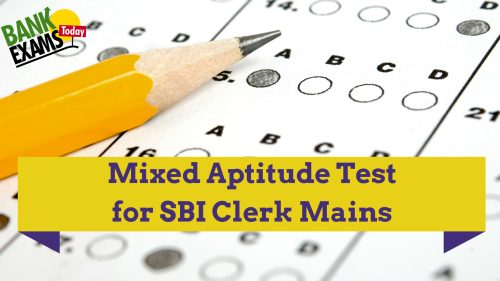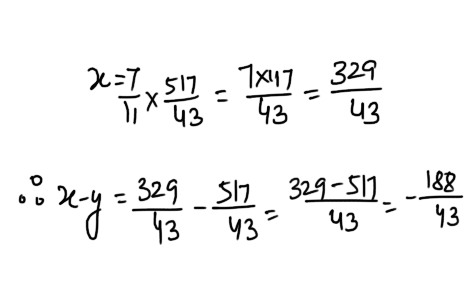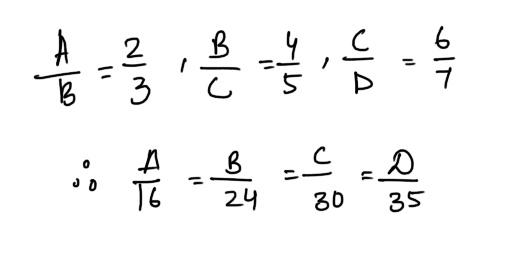# Mixed Aptitude Quiz for SBI Clerk Mains (Part - 7)#### Ques 1.

Madhuri invested Rs . 15,000 @ 10% per annum for one year .If the interest is compounded half yearly,then the amount received by Madhuri at the end of the year will be

(a) Rs 16,537.50
(b) Rs 16,500
(c) Rs. 16,525.50
(d) Rs 18, 150
= 15000 ( 1 + 5/100)2 = 15000 x 21/20 x 21/20
Rs . 16537.50.

#### Ques 2.

If 3x + 2y = 47 and 11x = 7y ,then what will be the value of x-y ?
(a) 4
(b) 6
(c) 7
(d) None of these
Ans 2.#### Ques 3.

If the volume of a sphere is divided by its surface area, then the result is 27 cm. The radius of the sphere is
(a) 9 cm
(b) 27 cm
(c) 81 cm
(d/) 243 cm
Ans 3. Let r be the radius of the sphere.#### Ques 4.

A can do a job in 6 days.While A and B together can do it in 2 Days .How long would it take B to do the job alone ?
(a) 3 days
(b) 4 days
(c) 5 days
(d) 6 days
Ans 4.  (A + B) 's  1 day's work = 1/2
A's 1 day's work = 1/6
,. . B's 1 day's work = 1/2 -1/6 = 3-1/6 = 2/6 =1/3
. .  B can do the job alone in 3 days

#### Ques 5.

If d is the H.C.F of two positive intergers a and b, then there exists two integers k and L such that
(a) a= kd + lb
(b) b = ka + ld
(c) d = ka + lb
(d) None of these
Ans 5.  b = ka + ld

#### Ques 6.

If A and B work together , they can finish a certain job in the three days. B and C together can finish the same job in six days whereas, A and C together can finish the same job in eight days. If three of them work on the job together,how many days will they take to finish that job ?
(a) More than three days
(b) Less than two days
(c) Less than three days
(d) None of the above
Ans 6. In 1 day both A and B can do
= 1/3rd  of the work
In 1 day both B and C can do
= 1/6th of the work
In 1 day both A and C can do
= 1/8 th of the work
In 1 day  a, B and C together can do work
= 2 ( 1/3 + 1/6 + 1/8) = 2 ( 8 + 4+3/24_ = 5/4
Sp, A, B and C finish the work in 4/5 day

#### Ques 7.

Rs 500 is invested at compound interest at the rate of 10% 2 years and Rs 800 is invested at the rate of 10% and compound interest is reckoned half yearly. If both the sum i s invested for a year, then what is the difference between interest ?
(a) Rs . 20
(b) Rs 27.
(c) Rs . 30
(d) None of these
Ans 7. According to the question , T = 2 years,
r = 10% , P = Rs 500#### Ques 8.

If A : B = 2 :3, B : C = 4 : 5 and C: D = 6 : 7, Then A : B : C: D is
(a) 18 : 24 : 30 : 35
(b) 16 : 24 : 30 : 35
(c) 16 : 22 : 30 : 35
(d) 16 : 24 : 15 : 35
Ans 8.#### Ques 9.

The L.C.M of two numbers is 14560 and their H.C.F . is 13. If one of them is 416, them the other is
(a) 460
(b) 455
(c) 450
(d) 445
Ans 9.  Since, the first no.  x second no. = L.C.m. x H.C.F
.  . The other no. = 14560 x 13/416= 455

#### Ques 10.

Gold is 19 times as heavy as water and and copper is 9 times as heavy as water. In what ratio should these be mixed to get alloy 16 times as heavy as heavy as water ?
(a) 4 : 5
(b) 5 :4
(c) 3 : 7
(d) 7 : 3
Ans 10.#### Ques 11.

At a game of billiards,A can give B 15 points in 60 and A can give C 20 in 60. How many can B give C in a game C in a game of 90 ?
(a) 30 points
(b) 20 points
(c) 10 points
(d) 12 points
Ans 11.